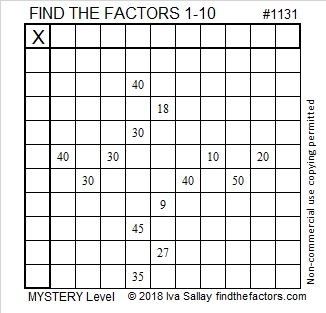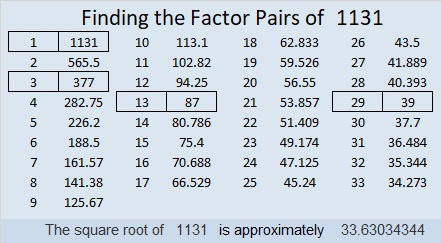# 1131 Mystery

You can’t know if this puzzle is easy or difficult by just looking at it. Give it a try. You might be surprised by your ability to do this puzzle!Print the puzzles or type the solution in this excel file: 10-factors-1121-1133

Here are some facts about the number 1131:

• 1131 is a composite number.
• Prime factorization: 1131 = 3 × 13 × 29
• The exponents in the prime factorization are 1, 1, and 1. Adding one to each and multiplying we get (1 + 1)(1 + 1)(1 + 1) = 2 × 2 × 2 = 8. Therefore 1131 has exactly 8 factors.
• Factors of 1131: 1, 3, 13, 29, 39, 87, 377, 1131
• Factor pairs: 1131 = 1 × 1131, 3 × 377, 13 × 87, or 29 × 39
• 1131 has no square factors that allow its square root to be simplified. √1131 ≈ 33.630341131 is the hypotenuse of FOUR Pythagorean triples:
405-1056-1131 which is 3 times (135-352-377)
435-1044-1131 which is (5-12-13) times 87
456-1035-1131 which is 3 times (152-345-377)
780-819-1131 which is (20-21-29) times 39

1131 looks interesting in a couple of other bases:
It’s 939 in BASE 11 because 9(11²) + 3(11) + 9(1) = 1131
234 in BASE 23 because 2(23²) + 3(23) + 4(1) = 1131